Courses

# NCERT Solutions - Solutions (Part - 2) Notes | EduRev

## Chemistry Class 12

Created by: Mohit Rajpoot

## Class 12 : NCERT Solutions - Solutions (Part - 2) Notes | EduRev

The document NCERT Solutions - Solutions (Part - 2) Notes | EduRev is a part of the Class 12 Course Chemistry Class 12.
All you need of Class 12 at this link: Class 12

Q.15. Define the following terms:
(a) Mole fraction
(b) Molality
(c) Molarity
(d) Mass percentage.
Ans.
(a) Mole fraction
The mole fraction of a component in a mixture is defined as the ratio of the number of moles of the component to the total number of moles of all the components in the mixture.
i.e.,
Mole fraction of a component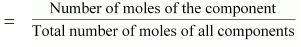Mole fraction is denoted by ‘x’.
If in a binary solution, the number of moles of the solute and the solvent are nA and nB respectively, then the mole fraction of the solute in the solution is given
by,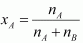Similarly, the mole fraction of the solvent in the solution is given as: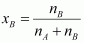(b) Molality
Molality (m) is defined as the number of moles of the solute per kilogram of the
solvent. It is expressed as:
Molality (m)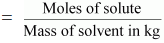(c) Molarity
Molarity (M) is defined as the number of moles of the solute dissolved in one Litre of the solution.
It is expressed as:
Molarity (M)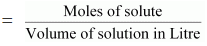(d) Mass percentage
The mass percentage of a component of a solution is defined as the mass of the solute in grams present in 100 g of the solution. It is expressed as:
Mass % of a component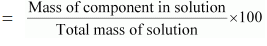Q.16. Concentrated nitric acid used in laboratory work is 68% nitric acid by mass in aqueous solution. What should be the molarity of such a sample of the acid if the density of the solution is 1.504 g mL−1?
Ans. Concentrated nitric acid used in laboratory work is 68% nitric acid by mass in an aqueous solution. This means that 68 g of nitric acid is dissolved in 100 g of the solution.
Molar mass of nitric acid (HNO3) = 1 × 1 + 1 × 14 + 3 × 16 = 63 g mol−1
Then, number of moles of HNO= 68/63 mol  = 1.079 mol
Given,
Density of solution = 1.504 g mL−1
Volume of 100 g solution = 100 / 1.504 mL
= 66.49 mL
= 66.49 × 10-3 mL
Molarity of solution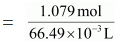= 16.23 M

Q.17. A solution of glucose in water is labelled as 10% w/w, what would be the molality and mole fraction of each component in the solution? If the density of solution is 1.2 g mL−1, then what shall be the molarity of the solution?
Ans. 10% w/w solution of glucose in water means that 10 g of glucose in present in 100 g of the solution i.e., 10 g of glucose is present in (100 − 10) g = 90 g of water.
Molar mass of glucose (C6H12O6) = 6 × 12 + 12 × 1 + 6 × 16 = 180 g mol−1
Then, number of moles of glucose  = 10/180 mol = 0.056 mol
Molality of solution = 0.056 mol / 0.09 kg = 0.62 m
Number of moles of water  = 90g / 18g mol-1 = 5 mol
Mole fraction of glucose (xg)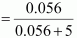= 0.011
And, mole fraction of water xw = 1 - xg
= 1 − 0.011 = 0.989
If the density of the solution is 1.2 g mL−1, then the volume of the 100 g solution can be given as: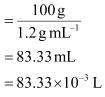∴ Molarity of the solution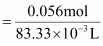= 0.67 M

Q.18. How many mL of 0.1 M HCl are required to react completely with 1 g mixture of Na2CO3 and NaHCO3 containing equimolar amounts of both?
Ans.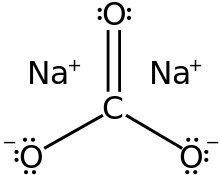Structure of Na2CO3

Let the amount of Na2CO3 in the mixture be x g.
Then, the amount of NaHCO3 in the mixture is (1 − x) g.
Molar mass of Na2CO3 = 2 × 23 + 1 × 12 + 3 × 16 = 106 g mol−1
Number of moles Na2CO= x / 106 mol
Molar mass of NaHCO3 = 1 × 23 + 1 × 12  + 3 × 16 = 84 g mol−1
Number of moles of NaHCO3  = 1 -x /84 mol
According to the question,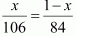⇒ 84x = 106 − 106x
⇒ 190x = 106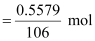x = 0.5579
Therefore, number of moles of Na2CO3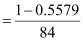= 0.0053 mol
And, number of moles of NaHCO3
= 0.0053 mol
HCl reacts with Na2CO3 and NaHCO3 according to the following equation.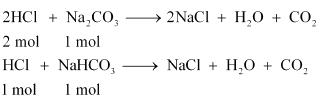1 mol of Na2CO3 reacts with 2 mol of HCl.
Therefore, 0.0053 mol of Na2CO3 reacts with 2 × 0.0053 mol = 0.0106 mol.
Similarly, 1 mol of NaHCO3 reacts with 1 mol of HCl.
Therefore, 0.0053 mol of NaHCO3 reacts with 0.0053 mol of HCl.
Total moles of HCl required = (0.0106 + 0.0053) mol
= 0.0159 mol
In 0.1 M of HCl,
0.1 mol of HCl is present in 1000 mL of the solution.
Therefore, 0.0159 mol of HCl is present in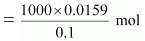= 159 mL of the solution
Hence, 159 mL of 0.1 M of HCl is required to react completely with 1 g mixture of Na2CO3 and NaHCO3, containing equimolar amounts of both.

Q.19. 100 g of liquid A (molar mass 140 g mol−1) was dissolved in 1000 g of liquid B (molar mass 180 g mol−1). The vapour pressure of pure liquid B was found to be 500 torr. Calculate the vapour pressure of pure liquid A and its vapour pressure in the solution if the total vapour pressure of the solution is 475 Torr.
Ans. Number of moles of liquid A,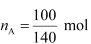= 0.714 mol
Number of moles of liquid B,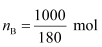= 5.556 mol
Then, mole fraction of A,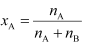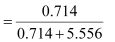= 0.114
And, mole fraction of B, xB = 1 − 0.114
= 0.886
Vapour pressure of pure liquid B, pbo = 500 torr
Therefore, vapour pressure of liquid B in the solution,
pb = pboxb
= 500 × 0.886
= 443 torr
Total vapour pressure of the solution, ptotal = 475 torr
Vapour pressure of liquid A in the solution,
pA = ptotalpB
= 475 − 443
= 32 torr
Now,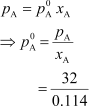= 280.7 torr
Hence, the vapour pressure of pure liquid A is 280.7 torr.

Q.20. An antifreeze solution is prepared from 222.6 g of ethylene glycol (C2H6O2) and 200 g of water. Calculate the molality of the solution. If the density of the solution is 1.072 g mL−1, then what shall be the molarity of the solution?
Ans. Molar mass of ethylene glycol [C2H4(OH)2] = 2 × 12 + 6 × 1 + 2 ×16 = 62 gmol−1
Number of moles of ethylene glycol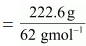= 3.59 mol
Therefore, molality of the solution = 3.59 MOL / 0.200 kg  = 17.95 m
Total mass of the solution = (222.6 + 200) g = 422.6 g
Given,
Density of the solution = 1.072 g mL−1
Volume of the solution = 422.6 g / 1.072g mL-1
= 394.22 mL
= 0.3942 × 10−3 L
⇒  Molarity of the solution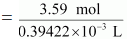= 9.11 M

Q.21. A sample of drinking water was found to be severely contaminated with chloroform (CHCl3) supposed to be a carcinogen. The level of contamination was 15 ppm (by mass):
(a) express this in percent by mass
(b) determine the molality of chloroform in the water sample.
Ans.
(a) 15 ppm (by mass) means 15 parts per million (106) of the solution.
Therefore, percent by mass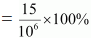= 1.5 × 10−3 %
(b) Molar mass of chloroform (CHCl3) = 1 × 12 + 1 × 1 + 3 × 35.5
= 119.5 g mol−1
Now, according to the question,
15 g of chloroform is present in 106 g of the solution.
i.e., 15 g of chloroform is present in (106 − 15) ≈ 106 g of water.
Molality of the solution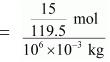= 1.26 × 10−4 m

Q.22. What role does the molecular interaction play in a solution of alcohol and water?
Ans. In pure alcohol and water, the molecules are held tightly by a strong hydrogen bonding. The interaction between the molecules of alcohol and water is weaker than alcohol−alcohol and water−water interactions. As a result, when alcohol and water are mixed, the intermolecular interactions become weaker and the molecules can easily escape. This increases the vapour pressure of the solution, which in turn lowers the boiling point of the resulting solution.

Q.23. Why do gases always tend to be less soluble in liquids as the temperature is raised?
Ans. Solubility of gases in liquids decreases with an increase in temperature. This is because dissolution of gases in liquids is an exothermic process.
Gas + Liquid  → Solution + Heat
Therefore, when the temperature is increased, heat is supplied and the equilibrium shifts backwards, thereby decreasing the solubility of gases.

Q.24. State Henry’s law and mention some important applications?
Ans. Henry’s law states that partial pressure of a gas in the vapour phase is proportional to the mole fraction of the gas in the solution. If p is the partial pressure of the gas in the vapour phase and x is the mole fraction of the gas, then Henry’s law can be expressed as:
p = Kx
Where,
KH is Henry’s law constant
Some important applications of Henry’s law are mentioned below.
(a) Bottles are sealed under high pressure to increase the solubility of CO2 in soft drinks and soda water.
(b) Henry’s law states that the solubility of gases increases with an increase in pressure. Therefore, when a scuba diver dives deep into the sea, the increased sea pressure causes the nitrogen present in air to dissolve in his blood in great amounts. As a result, when he comes back to the surface, the solubility of nitrogen again decreases and the dissolved gas is released, leading to the formation of nitrogen bubbles in the blood. This results in the blockage of capillaries and leads to a medical condition known as ‘bends’ or ‘decompression sickness’.
Hence, the oxygen tanks used by scuba divers are filled with air and diluted with helium to avoid bends.
(c) The concentration of oxygen is low in the blood and tissues of people living at high altitudes such as climbers. This is because at high altitudes, partial pressure of oxygen is less than that at ground level. Low-blood oxygen causes climbers to become weak and disables them from thinking clearly. These are symptoms of anoxia.

Offer running on EduRev: Apply code STAYHOME200 to get INR 200 off on our premium plan EduRev Infinity!

,

,

,

,

,

,

,

,

,

,

,

,

,

,

,

,

,

,

,

,

,

;